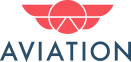## select SUM(CASE WHEN VoteTypeId = 2 THEN 1 ELSE 0 END)...

0

(click on this box to dismiss)Q&A for aircraft pilots, mechanics, and enthusiasts

``````select    SUM(CASE WHEN VoteTypeId = 2 THEN 1 ELSE 0 END) AS [Up Votes],
SUM(CASE WHEN VoteTypeId = 3 THEN 1 ELSE 0 END) AS [Down Votes],
(CAST(SUM(CASE WHEN VoteTypeId = 2 THEN 1 ELSE 0 END) AS float) / CAST(SUM(CASE WHEN VoteTypeId = 3 THEN 1 ELSE 0 END) AS float))Hold tight while we fetch your results